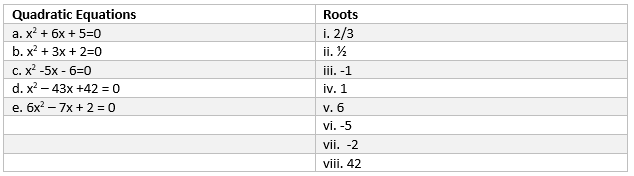# Quadratic equation class 10 extra questions

Given below are the class 10 quadratic equations extra questions
2. True and false
3. Multiple choice questions
4. Match the column

Question 1.
State which all quadratic equations have real roots, no real roots
1. $x^2 + x+7=0$
2. $3x^2 +6x+1=0$
3. $9x^2 +x +3=0$
4. $11x^2 -12x-1=0$
5. $-13x^2 +3x+7=0$
6. $2x^2 -6x+3=0$
7. $x- \frac {1}{x}-3=0$, x≠0
8. $-x^2 -2x-2=0$
Solution
Nature of roots of Quadratic equation
 S.no Condition Nature of roots 1 $b^2 -4ac > 0$ Two distinct real roots 2 $b^2 -4ac = 0$ One real root 3 $b^2 -4ac < 0$ No real roots
Real roots: :(b), (d) ,(e),(f),(g)
No real roots : (a) ,(c),(h)

## True and False

Question 2.
True or False statement
a. There are no reals roots of the quadratic equation $x^2+4x+5=0$
b. The roots of the equation $x^2-1=0$ are 1,-1
c. A quadratic equation can have at most 2 real roots
d. In a quadratic equation $ax^2 +bx+c=0$ ,if a and c are of same sign and b is zero ,the quadratic equation has real roots
e. In a quadratic equation $ax^2 +bx+c=0$ ,if a and c are of opposite sign, then quadratic equation will definitely have real roots
f. for k > 0,the quadratic equation $2x^2+6x-k=0$ will definitely have real roots
g. if the roots of the quadratic equation are rational, the coefficient of the term x will also be rational.
h. if the roots of the quadratic equation are irrational, the coefficient of the term x will also be irrational
i. Every quadratic equation will have rational roots
Solution
1. True
2. True
3. True
4. false
5. True
6. True
7. true
8. true
9. False

## Multiple choice Questions

Question 3.
Find a natural number whose square diminished by 84 is thrice the 8 more of given number
a. 21
b. 13
c.11
d. 12
Solution (d)
$x^2 -84=3(x+8)$
$x^2-3x-108=0$
x= 12 or -9

Question 4.
The roots of the quadratic equation
$x^2 +14x+40=0$ are
a.(4,10)
b.(-4,10)
c.(-4,-10)
d.(4,-10)
Solution
$x^2 +14x+40=0$
$x^2 + 4x + 10x +40=$
$x(x+4) + 10(x+4)=0$
$(x+4)(x+10)=0$
x=-4 or -10

Question 5.
The equation
$x^5 +x+20=0$
b. is not a quadratic equation
Solution
It is not a quadratic equation
Question 6.
The roots of the quadratic equation
$x^2+2x+5=0$
a. are real
b. are not real
Solution (b)
$b^2 - 4ac = 4 -20 =-16$

Question 7.
Which one of the following is not a quadratic equation?
a. $(x + 2)^2= 2(x + 3)$
b. $x^2 + 3x = (-1) (1 - 3x)^2$
c. $(x + 2) (x - 1) = x^2 - 2x - 5$
d. $x^3 - x^2 + 2x + 1 = (x + 1)^3$
Solution (b)
We can expand each of these expression and compare with $ax^2 + bx+x=0$.

Question 8.
Find the roots of
$6x^2- \sqrt {2}x - 2 = 0$ by the factorisation of the corresponding quadratic polynomial.
a. $-\frac {\sqrt {2}}{3},\frac {\sqrt {2}}{2}$
b. $-\frac {\sqrt {1}}{3},\frac {\sqrt {2}}{2}$
c. $-\frac {\sqrt {2}}{3},\frac {\sqrt {2}}{5}$
d. None of the these
Solution (b)
$6x^2- \sqrt {2}x - 2 = 0$
$6x^2 + 3 \sqrt {2}x -2\sqrt {2}x - 2 = 0$
$3x(2x - \sqrt {2}) - \sqrt {2}(2x - \sqrt {2})=0$
$(3x- \sqrt {2})(2x - \sqrt {2})=0$
or x = $-\frac {\sqrt {2}}{3},\frac {\sqrt {2}}{2}$

Question 9.
Had Ram scored 10 more marks in her mathematics test out of 30 marks, 9 times these marks would have been the square of her actual marks. How many marks did she get in the test?
a. 15 marks
b. 10 marks
c. 12 marks
d. 20 marks
Solution (b)
$9 (x +10) = x^2$
$x^2 - 9x - 90 = 0$
$(x + 6) (x -15) = 0$
Therefore, x = - 6 or x =15

## Match the Column

Question 10.
Match the roots with the quadratic equationsSolution a -> iii, vi
b-> iii, vii
c -> iii, v
d -> iv, viii
e. i, ii

Question 11.
Find the roots of the quadratic equation using factorization technique
a. $x^2-3x-10=0$
b. $x^2 -11x+30=0$
Solution
a.
$x^2-3x-10=0$
$x(x-5) +2(x-5)=0$
$(x+2)(x-5)=0$
So roots are x=-2 and 5
b. Roots are 5 and 6

Question 12.
Find the roots of the quadratic equation using square method
a. $x^2 +4x-5=0$
b. $2x^2-7x+3=0$
Solution
a.
$(x+ \frac {4}{2})^2 -(\frac {4}{2})^2 -5=0$
$(x+2)^2-9=0$
$(x+2)^2=9$
$x+2=\pm 3$
x=1 or -5
b.
$(x-\frac {7}{4})^2 -(\frac {7}{4})^2 +3/2=0$
$(x-\frac {7}{4})^2= \frac {49}{16} - \frac {3}{2}=0$
$(x-\frac {7}{4})^2=\frac {25}{16}$
$x-\frac {7}{4}=\pm \frac {5}{4}$
or
x=1/2 or 3

## Summary

This Class 10 Maths Extra Questions for Quadratic Equation along with solutions is prepared keeping in mind the latest syllabus of CBSE . This has been designed in a way to improve the academic performance of the students. If you find mistakes , please do provide the feedback on the mail.Go back to Class 10 Main Page using below links

### Practice Question

Question 1 What is $1 - \sqrt {3}$ ?
A) Non terminating repeating
B) Non terminating non repeating
C) Terminating
D) None of the above
Question 2 The volume of the largest right circular cone that can be cut out from a cube of edge 4.2 cm is?
A) 19.4 cm3
B) 12 cm3
C) 78.6 cm3
D) 58.2 cm3
Question 3 The sum of the first three terms of an AP is 33. If the product of the first and the third term exceeds the second term by 29, the AP is ?
A) 2 ,21,11
B) 1,10,19
C) -1 ,8,17
D) 2 ,11,20